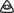#### Howdy, Stranger!

It looks like you're new here. If you want to get involved, click one of these buttons!

# "Averway" Shell script to average two Everway scores

This is more of a fun thought experiment based on the idea that a score of 3 is one normal person, a score of 4 is two normal people, a score of 5 is four normal people, a score of 2 is half as strong as a normal person etc.

I guess normally in a situation when you're comparing a pair elements (such as someones Integrity (Earth and Water), you'd just actually look at the two numbers separately. Resistant integrity along with receptive integrity.

Even if you would want to average two numbers, you'd do it directly on the Everway layer (3 and 5 = 4, unlike what this script would have it be, 4.32), or you'd look at the lowest of the two as sort of a "threshold" number, as per the Two Elements Test on p 109.

So this script was more of a thought experiment, just for fun. The "averway" of a 1 and a 7 becomes…6.02236781302845450829.

`#!/bin/sheverforward () { echo "2^(\${1}-3)"|bc -ql}average () { echo "(\$1 + \$2)/2"|bc -ql}everbackward () { echo "(l(\${1})/l(2))+3"|bc -ql}echo \$(everbackward \$(average \$(everforward \$1) \$(everforward \$2)))`

Usage
`\$ averway 1 76.02236781302845450829`

You can remove the `-3` and `+3` and the script will give the exact same results but run faster. They are only there to make the whole 3 → 1, 4 → 2, 2→0.5 thing work in case you'd want to use those functions separately.

One idea that I just came up with is… that instead of adding them together and dividing it by two, you'd multiply them together and then square root it. Like so:

`#!/bin/sheverforward () { echo "2^(\${1}-3)"|bc -ql}multivrage() { echo "sqrt((\$1 * \$2))"|bc -ql}everbackward () { echo "(l(\${1})/l(2))+3"|bc -ql}echo \$(everbackward \$(multivrage \$(everforward \$1) \$(everforward \$2)))`

That'd be way more better. A water 7 soul sister with a weak earth 1 body (a fourth as strong as a normal person) should have a combined score that reflects that; that takes the large number of the everforward(7) and makes it a quarter of the size before turning it back.

The funny thing that I found out is that… this give the exact same numbers as if we were to just average the numbers directly on the Everway layer. 1 and 7 becomes 4. 1 and 8 becomes 4.5. Any two numbers become their natural average.

Jonathan Tweet secret math genius…?! Illuminati confirmed…?• This is the difference between amounts and magnitudes, arithmetic means and geometric means. Anytime you're working in amount-space, arithmetic mean is likely natural ((2+8)*(1/2)=5). When you're working in magnitude-space, geometric mean is usually natural ((2*8)^(1/2)=4). And when you're given amounts of magnitudes, aka the logarithms of the quantity you actually care about, averaging the amounts should be the same as averaging the magnitudes.

In other words,

(A*B)^(1/2)=X
(logA+logB)*(1/2)=logX

aka

(a+b)*(1/2)=x
(2^a*2^b)^(1/2)=2^x
• That is the awesomest thing I've heard all weekend! I accidentally rediscovered geometric mean!

♥ thanx Guy!
• Thanks for that explanation Guy! Reading the post I was thinking, "Yes, this is definitely something I have done before," but my mathematizing days are so far behind me that I would never be able to actually explain it.